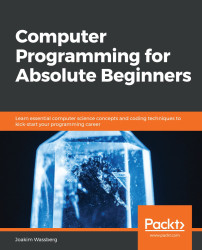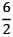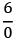•#### Computer Programming for Absolute Beginners#### Overview of this book

PrefaceSection 1: Introduction to Computer Programs and Computer ProgrammingFree Chapter
Chapter 1: Introduction to Computer ProgramsChapter 2: Introduction to Programming LanguagesChapter 3: Types of ApplicationsChapter 4: Software Projects and How We Organize Our CodeSection 2: Constructs of a Programming LanguageChapter 5: Sequence – The Basic Building Block of a Computer ProgramChapter 6: Working with Data – VariablesChapter 7: Program Control StructuresChapter 8: Understanding FunctionsChapter 9: When Things Go Wrong – Bugs and ExceptionsChapter 11: Programming Tools and MethodologiesSection 3: Best Practices for Writing High-Quality CodeChapter 12: Code QualityOther Books You May EnjoyAppendix A: How to Translate the Pseudocode into Real CodeAppendix B: Dictionary# Understanding types of software bugs

There are many different ways we can classify bugs. Here, we will look at some common types, see what they are, and see what they can look like.

## Arithmetic errors

Arithmetic bugs, as the name suggests, have to do with arithmetic operations. There are a few things we should look out for, as outlined in the following sections.

## Division by zero

One such thing is division by zero. This is not only related to computers as we can also never perform a division where the divisor is zero. In mathematics, dividing by zero has no meaning, because if we do, we will get 3. If we multiply 3 and 2, we will get 6 back. But if we take, there is no number we can multiply by zero to get back to 6.

This might seem simple enough, but sometimes, it happens anyway, especially when we are working with variables.

Let's assume that we have two variables that get their value somewhere in our application, like this:

```x = 3
y = 14```

Later on in the...